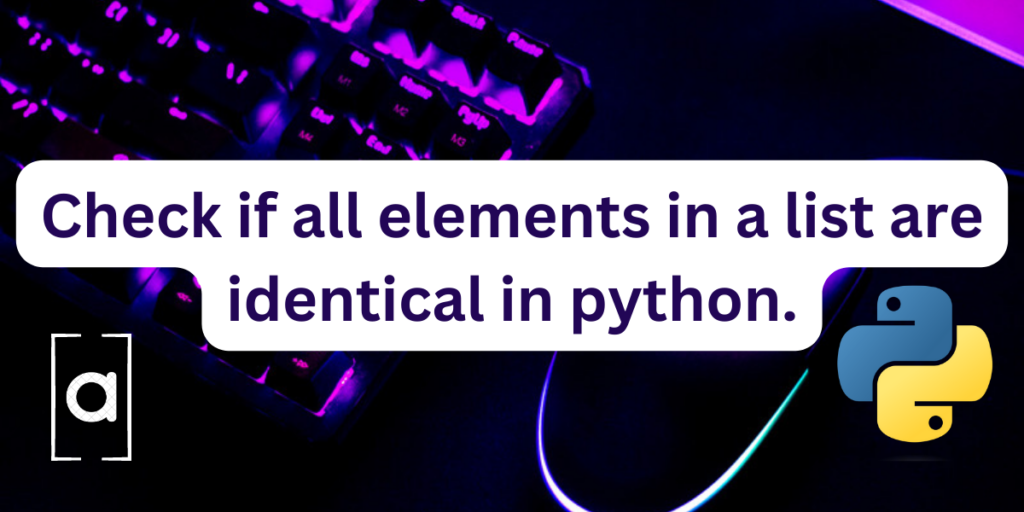# Check if all elements in a list are identical- 3 Easy methods.Python lists are objects that are similar to arrays or vectors in c and c++, used to store multiple values in one variable. Lists are one of the built in data types of python besides tuples, set and dictionaries. Lists are mutable in nature and can be changed later.

They are also ordered in nature meaning any new addition to an existing list will be located at the end of the list by default. Unlike tuples, lists can be modified by adding new elements or changing and removing existing elements. One can traverse through a list using loops like for and while. Some built-in functions are specific to lists in python and extremely useful.

## Properties of Python Lists.

• Lists are changeable, that is, they are mutable in nature.
• Lists allow duplicate elements.
• A list in python can contain elements of various data types.
• Lists are ordered in nature.
• The different elements in a list are separated by a comma.
• A list in python is defined inside a square bracket, `list =[]`
• Specific functions can be used to determine different properties of a list such as` len()` which determines the length or size of a list. The `append() `function is used to add elements to a list.
• An object can be explicitly assigned as a list by using the `list() `constructor.

## Checking the elements of a list

In order to locate or remove duplicate elements from a list, we need to compare its elements. This can be done in a number of ways. Lets look at some of them.

### Method 1: Manually checking the elements of a list

The first method defines a program to simply check whether the elements of a list are all the same or not.

```#assigning a list
L=[]
#size of the list
N=int(input("Enter the size of the list="))
#getting elements for the list
for i in range(N):
print("enter",i,"th element=",end='')
ip=input()
L.append(ip)
#displaying the original list
print("the original list is=",L)

#checking elements of list
first= L
#setting a counter
c=0
#checking the list
for i in L:
if i !=first:
print("the elements of the list are not all equal")
break
else:
c+=1
if(c==N):
print("the elements of the list are all equal")
```

Now, you will need to obtain user input and the output will depend on that input. My output was something as shown below:

```Enter the size of the list=4
enter 0 th element=hi
enter 1 th element=hi
enter 2 th element=hi
enter 3 th element=hi
the original list is= ['hi', 'hi', 'hi', 'hi']
the elements of the list are all equal
```

### Method 2: Using the itertools library

This is another way in which we can determine whether the elements of a list are all equal or not. Let’s look at the code.

```#importing required modules
from itertools import groupby

#assigning a list
L=[]
#size of the list
N=int(input("Enter the size of the list="))
#getting elements for the list
for i in range(N):
print("enter",i,"th element=",end='')
ip=input()
L.append(ip)
#displaying the original list
print("the original list is=",L)

#function to check whether the elements of a list are equal
def checkifallequal(L):
#using groupby
call = groupby(L)
return next(call, True) and not next(call, False)
#displaying the result
det=checkifallequal(L)
if(det=="True"):
print("the elements of the list are all equal.")
else:
print("the elements of the list are unequal.")
```

After taking user input, the output would be something like the following:

```Enter the size of the list=4
enter 0 th element=1
enter 1 th element=2
enter 2 th element=3
enter 3 th element=4
the original list is= ['1', '2', '3', '4']
the elements of the list are unequal.
```

Also read: Check if a List is Empty – 3 Easy Methods.

### Method 3: Using the length of the list to figure out the elements of the list

We can use the `len()` function to determine whether all the elements of a list are the same.

```#assigning a list
L=[]
#size of the list
N=int(input("Enter the size of the list="))
#getting elements for the list
for i in range(N):
print("enter",i,"th element=",end='')
ip=input()
L.append(ip)
#displaying the original list
print("the original list is=",L)
#check using the len() function
if([L]*len(L) == L):
print("all elements of the list are equal.")
else:
print("the elements of the list are not equal.")
```

The output of the code is:

```Enter the size of the list=5
enter 0 th element=this
enter 1 th element=is